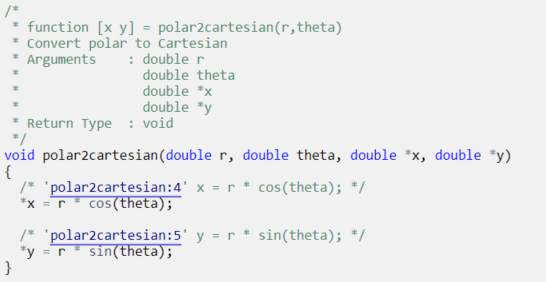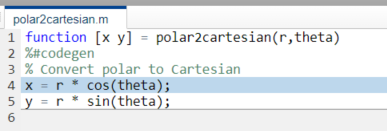## Include Comments in Generated C/C++ Code

#### Learning Objectives

This tutorial shows you how to generate code that includes:

• The function signature and function help text in the function banner.

• MATLAB® source code as comments with traceability tags. In the code generation report, the traceability tags link to the corresponding MATLAB source code.

#### Prerequisites

To complete this tutorial, you must have these products:

• MATLAB

• MATLAB Coder™

• Embedded Coder®

• C compiler

For a list of supported compilers, see Supported Compilers.

### Creating the MATLAB Source File

In a writable folder, create the MATLAB source file `polar2cartesian.m` that contains this code:

```function [x y] = polar2cartesian(r,theta) %#codegen % Convert polar to Cartesian x = r * cos(theta); y = r * sin(theta);```

### Configuring Build Parameters

Create a `coder.EmbeddedCodeConfig` code generation configuration object and set these properties to `true`:

• `GenerateComments` to allow comments in the generated code.

• `MATLABSourceComments` to generate MATLAB source code as comments with traceability tags. In the code generation report, the tags link to the corresponding MATLAB code. When this property is `true`, the code generator also produces the function signature in the function banner.

• `MATLABFcnDesc` to generate the function help text in the function banner.

```cfg = coder.config('lib', 'ecoder', true); cfg.GenerateComments = true; cfg.MATLABSourceComments = true; cfg.MATLABFcnDesc = true;```

### Generating the C Code

To generate C code, call the `codegen` function. Use these options:

• `-config` to pass in the code generation configuration object `cfg`.

• `-report` to create a code generation report.

• `-args` to specify the class, size, and complexity of the input parameters.

```codegen -config cfg -report polar2cartesian -args {0, 0} ```

`codegen` generates a C static library, `polar2cartesian.lib`, and C code in the `/codegen/lib/polar2cartesian` subfolder. Because you selected report generation, `codegen` provides a link to the report.

### Viewing the Generated C Code

View the generated code in the code generation report.

1. To open the code generation report, click ```View report```.

2. In the Generated Code pane, click `polar2cartesion.c`.

The generated code includes:

• The function signature and function help text in the function banner.

• Comments containing the MATLAB source code that corresponds to the generated C/C++ code. The comment includes a traceability tag that links to the original MATLAB code.The generated function banner also depends on the code generation template (CGT) file. With the default CGT, the code generator places information about the arguments in the function banner. You can customize the function banner by modifying the CGT. See Generate Custom File and Function Banners for C/C++ Code.

### Tracing the Generated Code to the MATLAB Code

Traceability tags provide information and links that help you to trace the generated code back to the original MATLAB code. For example, click the traceability tag that precedes the code ```x = r * cos(theta);```.The report opens `polar2cartesian.m` and highlights line 4.To view the MATLAB source code and generated C/C++ code next to each other and to interactively trace between them, in the report, click Trace Code. See Interactively Trace Between MATLAB Code and Generated C/C++ Code.